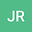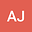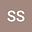New upper bounds for the forgotten index among bicyclic graphs
•••• +2Seyed Mahmoud Sheikholeslami
Author ProfileReza RasiThe forgotten topological index of a graph $G$, denoted by $F(G)$, is defined as the sum of weights $d(u)^{2}+d(v)^{2}$ over all edges $uv$ of $G$, where $d(u)$ denotes the degree of a vertex $u$. In this paper, we give sharp upper bounds of the F-index (forgotten topological index) over bicyclic graphs, in terms of the order and maximum degree.